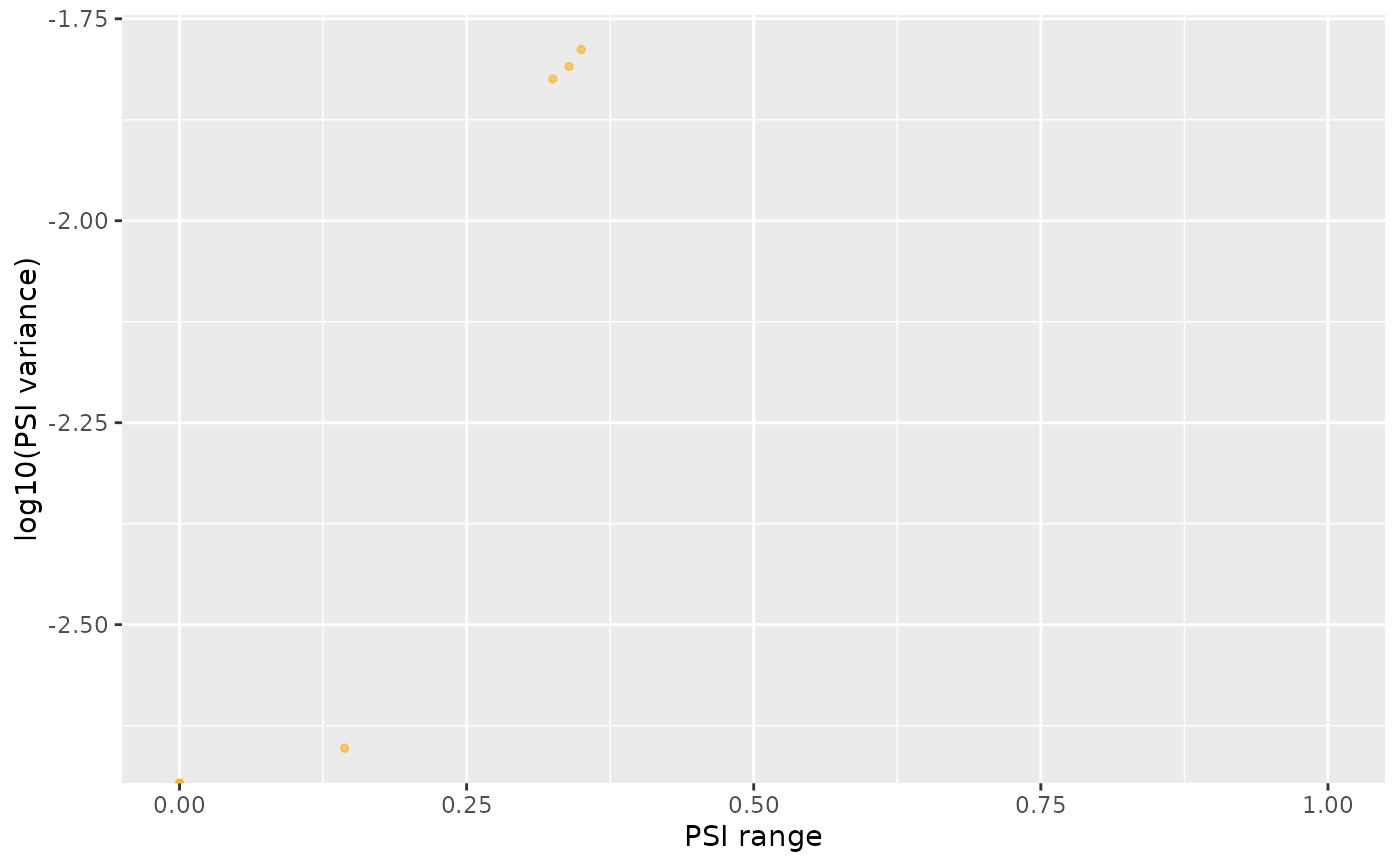Scatter plot to compare between the row-wise mean, median, variance or range from a data frame or matrix. Also supports transformations of those variables, such as log10(mean). If y = NULL, a density plot is rendered instead.

## Usage

plotRowStats(
data,
x,
y = NULL,
subset = NULL,
xmin = NULL,
xmax = NULL,
ymin = NULL,
ymax = NULL,
xlim = NULL,
ylim = NULL,
cache = NULL,
verbose = FALSE,
data2 = NULL,
legend = FALSE,
legendLabels = c("Original", "Highlighted")
)

## Arguments

data

Data frame or matrix containing samples per column and, for instance, gene or alternative splicing event per row

x, y

Character: statistic to calculate and display in the plot per row; choose between mean, median, var or range (or transformations of those variables, e.g. log10(var)); if y = NULL, the density of x will be plot instead

subset

Boolean or integer: data points to highlight

xmin, xmax, ymin, ymax

Numeric: minimum and maximum X and Y values to draw in the plot

xlim, ylim

Numeric: X and Y axis range

cache

List of summary statistics for data previously calculated to avoid repeating calculations (output also returns cache in attribute named cache with appropriate data)

verbose

Boolean: print messages of the steps performed

data2

Same as data argument but points in data2 are highlighted (unless data2 = NULL)

legend

Boolean: show legend?

legendLabels

Character: legend labels

## Value

Plot of data

Other functions for gene expression pre-processing: convertGeneIdentifiers(), filterGeneExpr(), normaliseGeneExpression(), plotGeneExprPerSample(), plotLibrarySize()

Other functions for PSI quantification: filterPSI(), getSplicingEventTypes(), listSplicingAnnotations(), loadAnnotation(), quantifySplicing()

## Examples

library(ggplot2)

# Plotting gene expression data
plotRowStats(geneExpr, "mean", "var^(1/4)") +
ggtitle("Mean-variance plot") +
labs(y="Square Root of the Standard Deviation")# Plotting alternative splicing quantification
psi <- quantifySplicing(annot, junctionQuant, eventType=c("SE", "MXE"))
#> Using 3 of 3 events (100%) whose junctions are present in junction quantification data...
#>   |                                        |   0%
|========                                |  20%
|================                        |  40%
|========================                |  60%
|================================        |  80%
|========================================| 100%

#> Using 3 of 3 events (100%) whose junctions are present in junction quantification data...
#>   |                                        |   0%
|========                                |  20%
|================                        |  40%
|========================                |  60%
|================================        |  80%
|========================================| 100%

medianVar <- plotRowStats(psi, x="median", y="var", xlim=c(0, 1)) +
labs(x="Median PSI", y="PSI variance")
medianVarrangeVar  <- plotRowStats(psi, x="range", y="log10(var)", xlim=c(0, 1)) +
labs(x="PSI range", y="log10(PSI variance)")
rangeVar